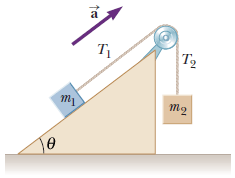# Torque and a pulleyI'm supposed to find, symbolically, what the moment of inertia of the pulley is using $T_1, T_2, r,$ and $a$. Obviously the base equation would be the sum of the torques equals $Iα$.

I have the answer, but I don't get one part. Why exactly is the sum of the torques $T_2r - T_1r$? It's obvious that the net torque is $\tau_2-\tau_1$, but why is the torque using the tension equal to $T_1r$ and $T_2r$?

• $r$ would be from the center of the axle for the pulley to the center of the rope in the pulley channel.
– MaxW
Apr 12, 2016 at 1:48
• Please see our guide on writing good titles.
– user10851
Apr 12, 2016 at 3:01
• what do you want to ask?
– Anni
Apr 12, 2016 at 8:42
• This is nicely discussed in this article.
– BoLe
Jun 19, 2016 at 11:04

The torque that is applied to the pulley is created by friction between string and pulley. String has no mass but it has acceleration. So the force and torque that are applied to it must be zero. $$\sum{\tau}=I\alpha\;,\,\;I=0\;\Rightarrow\sum{\tau}=0$$ Free body diagram of string is shown below:If we write $\sum{\tau}$ about center of pulley, then we have:$$\sum{\tau}=T_2r-\int_0^\theta r\mathrm dF_f-T_1r=0$$ Consider that $\vec N=\int_0^\theta \mathrm d\vec N$ passes from center of pulley and its torque is zero (all of $\color{red}{\mathrm dN}$s pass from center of pulley). So, we have:$$\int_0^\theta r\mathrm dF_f=\left(T_2-T_1\right)r$$ And according to the third law of newton, this torque is applied to pulley in the inverse direction.

Torque is force times the distance from the rotation point. (More specifically, it is the cross product.) The distance from the rotation point is just $r$, and the forces are $T_1$ and $T_2$

So,

$$\tau_1 = T_1r$$

and

$$\tau_2 = T_2r$$